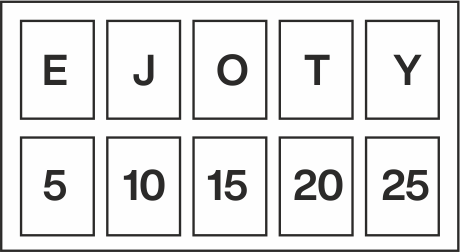# SERIES

## NUMBER SERIES:

Identify the pattern and apply it to the number before / after the missing number in the series to get the right answer.

## NUMBER SERIES PATTERNS:

1. Prime Numbers: Given below is a series of prime numbers.
Example: 11, 13, 17, 19...
2. Squares / Cubes of numbers: Given below are example of series of perfect square or cube roots.
Example:
Square of number, 92 = 81, 102 = 100, 112 = 121, 122 = 144, 132 = 169.....
Cube of number. 43 = 64. 53 = 125. 63 = 216, 73 = 343.....
3. Patterns in differences: Calculate the difference between the given numbers in the series.
Example : 2, 5, 8, 11, 14... (The difference between the numbers here is 3, hence the next number will be 17)
4. Geometric Series: It is a case where each successive number in the series is obtained by multiplying or dividing the previous number by a fixed number.
Example: 5, 45, 405, 3645
5. Odd One Out: When one number is not a part of a series, for example: 5, 10, 12, 15, 20... (Here all numbers except, 12 are multiples of 5)
6. n2-1 Series: Example: 0, 3, 8, 15, 24, 35, 48, ………
7. n2+1 Series: Example: 2, 5, 10, 17, 26, 37, ........., 65.
8. The middle digit is the sum of the other two digits. Example: 165, 121, etc

## ALPHABET SERIES

While solving the problems based on alphabets, please remember the exact position of every alphabet in forward as well as in backward or reverse orders, as given below:
Positions of letters in forward alphabetical order: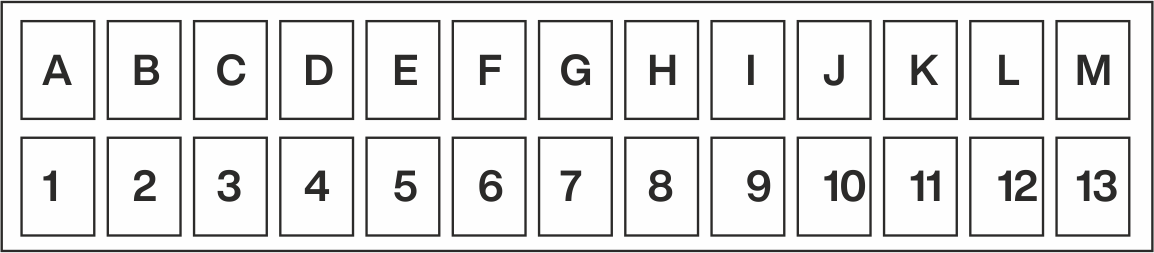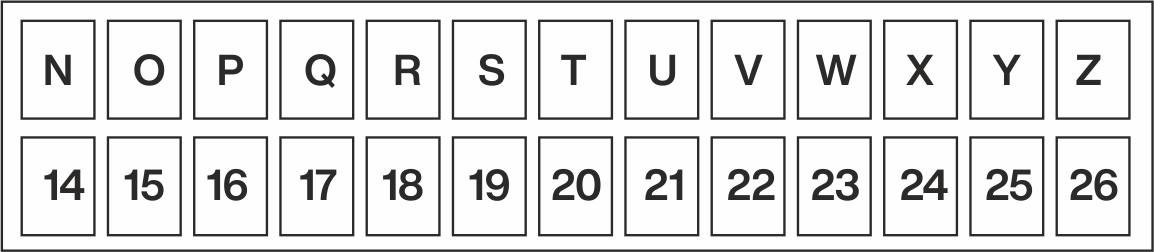Positions of letters in backward or reverse alphabetical order: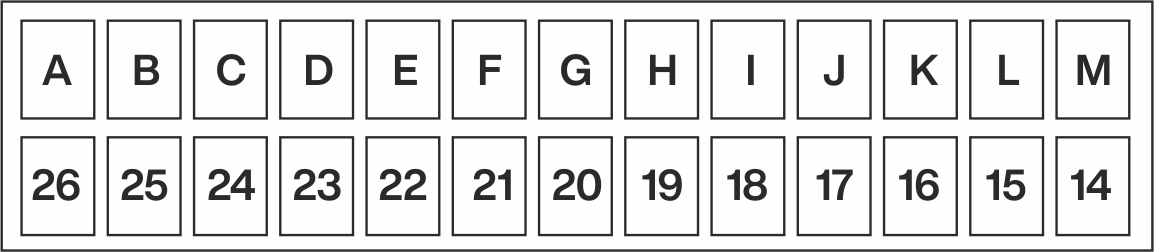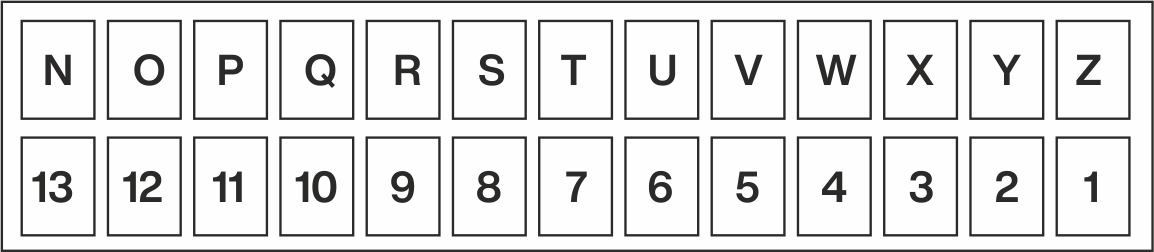Here, the mth element counted from left to right of a series of x characters is equal to (x + 1 - m)th element counted from right to left of that series.

## Example :

Counting from left to right, find the position of J in the above given series.
Position of "J" in the English alphabet from left to right is 10. Thus m = 10
Position of J in the above given series from right to left would be (26 + 1 - 10) = 17
The first half of the alphabet series is from A to M., i.e., 1 - 13 and the second half is from N to Z. i.e., 14 - 26

## Tricks:

I. EJOTY: You can locate the position of the letters easily and quickly (i.e. from left to right), using the trick called EJOTY.